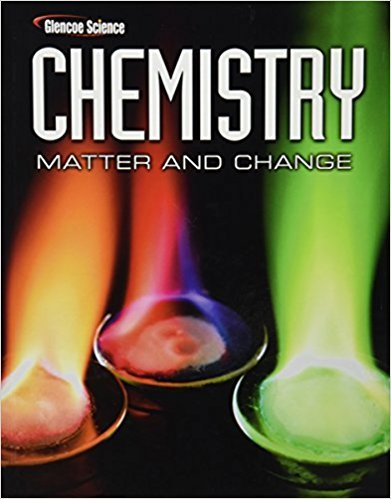×
Log in to StudySoup
Get Full Access to Chemistry: Matter & Change - 1 Edition - Chapter 3 - Problem 3
Join StudySoup for FREE
Get Full Access to Chemistry: Matter & Change - 1 Edition - Chapter 3 - Problem 3

Already have an account? Login here
×
Reset your password

# Classify each of the following as a physical or a chemicalISBN: 9780078746376 131

## Solution for problem 3 Chapter 3

Chemistry: Matter & Change | 1st Edition

• Textbook Solutions
• 2901 Step-by-step solutions solved by professors and subject experts
• Get 24/7 help from StudySoup virtual teaching assistantsChemistry: Matter & Change | 1st Edition

4 5 1 295 Reviews
15
0
Problem 3

Classify each of the following as a physical or a chemical property. a. Iron and oxygen form rust. b. Iron is more dense than aluminum. c. Magnesium burns brightly when ignited. d. Oil and water do not mix. e. Mercury melts at 39C.

Step-by-Step Solution:
Step 1 of 3

The bigger Ka is, the stronger the acid is, and the less basic that its anion is. For all strong acids, the anion is neutral, but for weak acids, the anion is basic. Ex. Perchlorate and sulfate are neutral anions, but fluoride and cyanide are basic anions. If a salt contains a basic anion, the salt will form a basic solution when dissolved in water. Ex. Sodium acetate, potassium fluoride. Useful equations for pH problems: −¿ OH ¿ ¿ ¿ +¿ H ¿ ¿ ¿ pKa+pKb=14 Sample problem: You have a solution of 0.1 M HCl and 0.1 M HF. Calculate the pH. The HCl will dissociate completely, so that the concentration of the free hydrogen ions will be equal to the HCl concentration. The HF does not dissociate completely. It dissociates according to the following equation:

Step 2 of 3

Step 3 of 3

##### ISBN: 9780078746376

Since the solution to 3 from 3 chapter was answered, more than 331 students have viewed the full step-by-step answer. The full step-by-step solution to problem: 3 from chapter: 3 was answered by , our top Chemistry solution expert on 11/10/17, 05:56PM. Chemistry: Matter & Change was written by and is associated to the ISBN: 9780078746376. This full solution covers the following key subjects: . This expansive textbook survival guide covers 24 chapters, and 2831 solutions. The answer to “Classify each of the following as a physical or a chemical property. a. Iron and oxygen form rust. b. Iron is more dense than aluminum. c. Magnesium burns brightly when ignited. d. Oil and water do not mix. e. Mercury melts at 39C.” is broken down into a number of easy to follow steps, and 43 words. This textbook survival guide was created for the textbook: Chemistry: Matter & Change, edition: 1.

Unlock Textbook Solution

Enter your email below to unlock your verified solution to:

Classify each of the following as a physical or a chemical